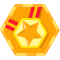直接通过Runtime进行调用

``````public class InvokeByRuntime {
/**
* @param args
* @throws IOException
* @throws InterruptedException
*/
public static void main(String[] args) throws IOException， InterruptedException {
String exe = "python";
String command = "D:\\calculator_simple.py";
String num1 = "1";
String num2 = "2";
String[] cmdArr = new String[] {exe， command， num1， num2};
Process process = Runtime.getRuntime().exec(cmdArr);
InputStream is = process.getInputStream();
DataInputStream dis = new DataInputStream(is);
process.waitFor();
System.out.println(str);
}
}``````

``3``

calculator_simple.py:

``````# coding=utf-8
from sys import argv

num1 = argv
num2 = argv
sum = int(num1) + int(num2)
print sum``````

通过Jython调用

1. 什么是Jython

Jython主页：http://www.jython.org/currentdocs.html

Python其实只是一个语言规范，它存在多个不同语言实现的版本。具体来说，目前Python语言存在如下几个具体实现：
（1）CPython：CPython是标准Python，也是其他Python编译器的参考实现。通常提到“Python”一词，都是指CPython。CPython由C编写，将Python源码编译成CPython字节码，由虚拟机解释执行。没有用到JIT等技术，垃圾回收方面采用的是引用计数。
（2）Jython：Jython是在JVM上实现的Python，由Java编写。Jython将Python源码编译成JVM字节码，由JVM执行对应的字节码。因此能很好的与JVM集成，比如利用JVM的垃圾回收和JIT，直接导入并调用JVM上其他语言编写的库和函数。
（3）IronPython：IronPython与Jython类似，所不同的是IronPython在CLR上实现的Python，即面向.NET平台，由C#编写。IronPython将源码编译成TODO CLR，同样能很好的与.NET平台集成。即与Jython相同，可以利用.NET框架的JIT、垃圾回收等功能，能导入并调用.NET上其他语言编写的库和函数。IronPython默认使用Unicode字符串。
（4）PyPy：这里说的PyPy是指使用RPython实现，利用Tracing JIT技术实现的Python，而不是RPython工具链。PyPy可以选择多种垃圾回收方式，如标记清除、标记压缩、分代等。
（5）Pyston：Pyston由Dropbox开发，使用C++11编写，采用Method-at-a-time-JIT和Mark Sweep——Stop the World的GC技术。Pyston使用类似JavaScript V8那样的多层编译，其中也用到了LLVM来优化代码。

Jython原本叫做JPython，于1997年由Jim Hugunin创建，后来在1999年2.0版本发布的时候由Barry Warsaw更名为Jython，在这里我们就不再深究为什么要把JPython更名为Jython的原因了。注意： Jython从2.0版本开始就与CPython的版本保持一致，即：Jython 2.7与CPython 2.7保持对应。

3. 如何使用Jython

3.1 安装Jython``java -jar jython-installer-\${version}.jar``

``````> jython
Jython 2.7.0 (default:9987c746f838， Apr 29 2015， 02:25:11)
[Java HotSpot(TM) 64-Bit Server VM (Oracle Corporation)] on java1.8.0_121
>>> print("hello，world")
hello，world
>>>``````

``````> jython helloworld.py
hello，world``````

helloworld.py:

``````import sys

print("hello，world")``````

``````<dependency>
<groupId>org.python</groupId>
<artifactId>jython</artifactId>
<version>2.7.0</version>
</dependency>``````

3.2 Java调用Python程序实践

Java通过Jython API调用Python程序，有几种用法：
（1）在Java中执行Python语句，相当于在Java中嵌入了Python程序，这种用法不常见，也没有太大的实际意义。

``````public static void main(String[] args) {
System.setProperty("python.home"， "D:\\jython2.7.0");
PythonInterpreter interp = new PythonInterpreter();
// 执行Python程序语句
interp.exec("import sys");
interp.set("a"， new PyInteger(42));
interp.exec("print a");
interp.exec("x = 2+2");
PyObject x = interp.get("x");
System.out.println("x: " + x);
}``````

``````42
x: 4``````

（2）在Java中简单调用Python程序，不需要传递参数，也不需要获取返回值。

``````public static void main(String[] args) throws IOException {
System.setProperty("python.home"， "D:\\jython2.7.0");
String python = "D:\\simple_python.py";
PythonInterpreter interp = new PythonInterpreter();
interp.execfile(python);
interp.cleanup();
interp.close();
}``````

simple_python.py:

``````# coding=utf-8
print("Do simple thing in Python")
print("输出中文")``````

（3）在Java中单向调用Python程序中的方法，需要传递参数，并接收返回值。Python既支持面向函数式编程，也支持面向对象编程。因此，调用Python程序中的方法也分别以面向函数式编程和面向对象式编程进行说明。

``````public static void main(String[] args) throws IOException {
System.setProperty("python.home"， "D:\\jython2.7.0");

// 1. Python面向函数式编程: 在Java中调用Python函数
String pythonFunc = "D:\\calculator_func.py";

PythonInterpreter pi1 = new PythonInterpreter();
// 加载python程序
pi1.execfile(pythonFunc);
// 调用Python程序中的函数
PyFunction pyf = pi1.get("power", PyFunction.class);
PyObject dddRes = pyf.__call__(Py.newInteger(2), Py.newInteger(3));
System.out.println(dddRes);
pi1.cleanup();
pi1.close();

// 2. 面向对象式编程: 在Java中调用Python对象实例的方法
String pythonClass = "D:\\calculator_clazz.py";
// python对象名
String pythonObjName = "cal";
// python类名
String pythonClazzName = "Calculator";
PythonInterpreter pi2 = new PythonInterpreter();
// 加载python程序
pi2.execfile(pythonClass);
// 实例化python对象
pi2.exec(pythonObjName + "=" + pythonClazzName + "()");
// 获取实例化的python对象
PyObject pyObj = pi2.get(pythonObjName);
// 调用python对象方法,传递参数并接收返回值
PyObject result = pyObj.invoke("power", new PyObject[] {Py.newInteger(2), Py.newInteger(3)});
double power = Py.py2double(result);
System.out.println(power);

pi2.cleanup();
pi2.close();
}``````

``````8.0
8.0``````

calculator_func.py：

``````# coding=utf-8
import math

# 面向函数式编程
def power(x, y):
return math.pow(x, y)``````

calculator_clazz.py：

``````# coding=utf-8
import math

# 面向对象编程
class Calculator(object):

# 计算x的y次方
def power(self, x, y):
return math.pow(x,y)``````

（4）高级调用，也是在Java中调用Python程序最常见的用法：Python程序可以实现Java接口，在Python中也可以调用Java方法。

``````public static void main(String[] args) throws IOException {
System.setProperty("python.home"， "D:\\jython2.7.0");

// Python程序路径
String python = "D:\\python\\fruit_controller.py";
// Python实例对象名
String pyObjName = "pyController";
// Python类名
String pyClazzName = "FruitController";

Fruit apple = new Apple();
Fruit orange = new Orange();

PythonInterpreter interpreter = new PythonInterpreter();
// 如果在Python程序中引用了第三方库,需要将这些被引用的第三方库所在路径添加到系统环境变量中
// 否则,在执行Python程序时将会报错: ImportError: No module named xxx
PySystemState sys = interpreter.getSystemState();

// 加载Python程序
interpreter.execfile(python);
// 实例 Python对象
interpreter.exec(pyObjName + "=" + pyClazzName + "()");

// 1.在Java中获取Python对象,并将Python对象转换为Java对象
// 为什么能够转换? 因为Python类实现了Java接口,通过转换后的Java对象只能调用接口中定义的方法
GroovyController controller = (GroovyController) interpreter.get(pyObjName).__tojava__(GroovyController.class);
controller.controllFruit(apple);
controller.controllFruit(orange);

// 2.在Java直接通过Python对象调用其方法
// 既可以调用实现的Java接口方法,也可以调用Python类自定义的方法
PyObject pyObject = interpreter.get(pyObjName);
pyObject.invoke("controllFruit", Py.java2py(apple));
pyObject.invoke("controllFruit", Py.java2py(orange));
pyObject.invoke("printFruit", Py.java2py(apple));
pyObject.invoke("printFruit", Py.java2py(orange));

// 3.在Java中获取Python类进行实例化对象: 没有事先创建 Python对象
PyObject pyClass = interpreter.get("FruitController");
PyObject pyObj = pyClass.__call__();
pyObj.invoke("controllFruit", Py.java2py(apple));
pyObj.invoke("controllFruit", Py.java2py(orange));

PyObject power = pyObj.invoke("power", new PyObject[] {Py.newInteger(2), Py.newInteger(3)});
if(power != null) {
double p = Py.py2double(power);
System.out.println(p);
}

interpreter.cleanup();
interpreter.close();
}``````

``````Show: I am a java apple.
controllFruit Python Apple
controllFruit END
Show: I am a java orange.
controllFruit Python Orange
controllFruit END
Show: I am a java apple.
controllFruit Python Apple
controllFruit END
Show: I am a java orange.
controllFruit Python Orange
controllFruit END
Show: I am a java apple.
printFruit Python Apple
printFruit END
Show: I am a java orange.
printFruit Python Orange
printFruit END
Show: I am a java apple.
controllFruit Python Apple
controllFruit END
Show: I am a java orange.
controllFruit Python Orange
controllFruit END
8.0``````

fruit_controller.py：

``````# coding=utf-8

from calculator_clazz import Calculator
from java.lang import String
from org.test.inter import GroovyController
from org.test.inter import Fruit

# 在Python中实现Java接口: org.test.inter.GroovyController
class FruitController(GroovyController):

# 实现接口方法
def controllFruit(self, fruit):
# 在Python中调用Java对象方法
fruit.show()

if(fruit.getType() == "apple"):
print ("controllFruit Python Apple")

if(fruit.getType() == "orange"):
print ("controllFruit Python Orange")

print ("controllFruit END")

# 自定义新方法
def printFruit(self, fruit):
fruit.show()

if(fruit.getType() == "apple"):
print ("printFruit Python Apple")

if(fruit.getType() == "orange"):
print ("printFruit Python Orange")

print ("printFruit END")

# 引用第三方python程序
def power(self, x, y):
cal = Calculator()
return cal.power(x, y)``````

Java接口和实现类：

``````// 该接口用于在Python中实现
public interface GroovyController {
public void controllFruit(Fruit fruit);
}

// 在Java中使用的接口
public interface Fruit {
public String getName();
public String getType();
public void show();
}

// Apple
public class Apple implements Fruit {
public String getName() {
return "java apple";
}

public String getType() {
return "apple";
}

public void show() {
System.out.println("Show: I am a java apple.");
}
}

// Orange
public class Orange implements Fruit {
public String getName() {
return "ava orange";
}

public String getType() {
return "orange";
}

public void show() {
System.out.println("Show: I am a java orange.");
}
}``````

``Failed to install '': java.nio.charset.UnsupportedCharsetException: cp0``

总结

【参考】
http://tonl.iteye.com/blog/1918245 Java调用Python
http://blog.csdn.net/supermig/article/details/24005585 Learning Python -- Java 通过JyThon调用Python实现的规则
http://blog.csdn.net/hpp1314520/article/details/72854011 java 利用Runtime.getRuntime().exec()调用python脚本并传参
http://blog.csdn.net/xingjiarong/article/details/49424253 java调用python方法总结
https://zh.wikipedia.org/wiki/Jython Jython
http://lib.csdn.net/article/python/1654 Jython的安装及简单例子
https://coolshell.cn/articles/2631.html 五大基于JVM的脚本语言
http://python.jobbole.com/82703/ 各种 Python 实现的简单介绍与比较
https://www.oschina.net/translate/why-are-there-so-many-pythons 为什么有这么多 Python?榴莲黑芝麻糊Anoyi
2018/09/29
0
0
（转载）Python 的 JPype 模块调用 Jar 包

Python 的 JPype 模块调用 Jar 包 import os.path startJVM("F:/Java/jdk1.8.0_45/jre/bin/server/jvm.dll", "-ea") java.lang.System.out.println("hello World") shutdownJVM() import jp......

2018/03/21
0
0
Py4J —— Python 和 Java 的互调接口

Py4J 使得 Python 程序可以利用 Python 解释器直接调用Java虚拟机中的 Java 对象，也可以让 Java 调用 Python 对象，有点像 Python 版的 JNI。...

2010/10/02
134
0
Java 帝国对 Python 的渗透能成功吗？哈哈

04/21
0
1

2018/07/27
134
1

15分钟前
1
0
python from import与import as 的含义

from os import makedirs, unlink, sep #从os包中引入 makedirs.unlink,sep类 from os.path import dirname, exists, isdir, splitext 从 os包中的path类中引入 dirmame exists 等方法 impo......

dillonxiao
15分钟前
1
0
【转】URL最大长度问题

ZhangLG
17分钟前
0
0
Segment段

CurrentHashMap和HashMap相比支持并发操作，整个CurrentHashMap是由一个个的Segment组成的，也是就是常说的分段锁 Segment继承了重入锁ReentrantLock来进行加锁， 可以简单的把CurrentHashMa...

19分钟前
0
0
JS Date 自定义格式化方法

JS Date 自定义格式化方法 Date 时间对象 快速 自定义格式化 定义方法 // 自定义格式化方法Date.prototype.format = function(fmt) { var o = { "M+" : this.getMonth()+1, ......

DrChenXX
24分钟前
0
0